# Using jti

library(jti)
#>
#> Attaching package: 'jti'
#> The following object is masked from 'package:methods':
#>
#>     initialize
library(igraph)
#>
#> Attaching package: 'igraph'
#> The following objects are masked from 'package:stats':
#>
#>     decompose, spectrum
#> The following object is masked from 'package:base':
#>
#>     union

## On Graphical Models

The family of graphical models is vast and includes many different models. jti handles Bayesian networks and decomposable undirected graphical models. Undirected graphical models are also known as Markov random fields (MRFs). Decomposability is a property ensuring a closed form of the maximum likelihood parameters. Graphical models enjoy the property that conditional independencies can be read off from a graph consisting of nodes, representing the random variables. In Bayesian networks, edges are directed from a node to another and represent directed connections. In a MRF the edges are undirected and these should be regarded as associations between pairs of nodes in a broad sense. A Bayesian network is specified through a collection of conditional probability tables (CPTs) as we outline in the following and a MRF is specified through tables described by the cliques of the graph. Graphical models are used in a diverse range of applications, e.g., forensic identification problems, traffic monitoring, automated general medical diagnosis and risk analysis . Finally, we mention that the word posterior inference within the realm of graphical models is synonymous with estimating conditional probabilities.

Let $$p$$ be a discrete probability mass function of a random vector $$X = (X_{v} \mid v \in V)$$ where $$V$$ is a set of labels. The state space of $$X_{v}$$ is denoted $$I_{v}$$ and the state space of $$X$$ is then given by $$I = \times_{v\in V} I_{v}$$. A realized value $$x = (x_{v})_{v\in V}$$ is called a cell. Given a subset $$A$$ of $$V$$, the A-marginal cell of $$x$$ is the vector, $$x_{A} = (x_v)_{v\in A}$$, with state space $$I_{A} = \times_{v\in A} I_{v}$$. A Bayesian Network can be defined as a directed acyclic graph (DAG), for which each node represents a random variable together with a joint probability of the form

\begin{align} p(x) = \prod_{v\in V} p(x_{v} \mid x_{pa(v)}), \end{align}

where $$x_{pa(v)}$$ denotes the parents of $$x_v$$; i.e. the set of nodes with an arrow pointing towards $$x_v$$ in the DAG. Also, $$x_v$$ is a child of the variables $$x_{pa(v)}$$. Notice, that $$p(x_{v} \mid x_{pa(v)})$$ has domain $$I_{v} \times I_{pa(v)}$$.

## Setting up the network

el <- matrix(c(
"A", "T",
"T", "E",
"S", "L",
"S", "B",
"L", "E",
"E", "X",
"E", "D",
"B", "D"),
nc = 2,
byrow = TRUE
)

g <- igraph::graph_from_edgelist(el)
plot(g)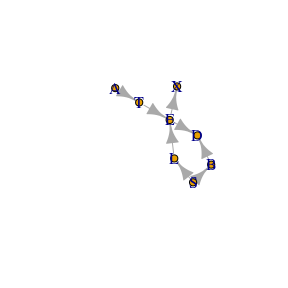We use the asia data; see the man page (?asia)

### Compilation

Checking and conversion

cl <- cpt_list(asia, g)
cl
#>  List of CPTs
#>  -------------------------
#>   P( A )
#>   P( T | A )
#>   P( E | T, L )
#>   P( S )
#>   P( L | S )
#>   P( B | S )
#>   P( X | E )
#>   P( D | E, B )
#>
#>   <bn, cpt_list, list>
#>  -------------------------

Compilation

cp <- compile(cl)
cp
#>  Compiled network  (cpts initialized)
#>  ------------------------------------
#>   Nodes: 8
#>   Cliques: 6
#>    - max: 3
#>    - min: 2
#>    - avg: 2.67
#>   <bn, charge, list>
#>  ------------------------------------
# plot(get_graph(cp)) # Should give the same as plot(g)

After the network has been compiled, the graph has been triangulated and moralized. Furthermore, all conditional probability tables (CPTs) has been designated to one of the cliques (in the triangulated and moralized graph).

### Example 1: sum-flow without evidence

jt1 <- jt(cp)
jt1
#>  Junction Tree
#>  -------------------------
#>   Propagated: full
#>   Flow: sum
#>   Cliques: 6
#>    - max: 3
#>    - min: 2
#>    - avg: 2.67
#>   <jt, list>
#>  -------------------------
plot(jt1)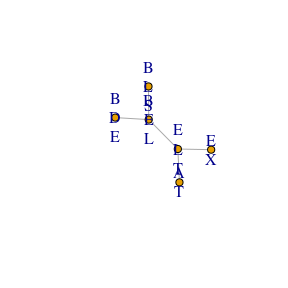Query probabilities:

query_belief(jt1, c("E", "L", "T"))
#> $E #> E #> n y #> 0.9257808 0.0742192 #> #>$L
#> L
#>     n     y
#> 0.934 0.066
#>
#> $T #> T #> n y #> 0.9912 0.0088 query_belief(jt1, c("B", "D", "E"), type = "joint") #> , , B = y #> #> E #> D n y #> y 0.36261346 0.041523361 #> n 0.09856873 0.007094444 #> #> , , B = n #> #> E #> D n y #> y 0.04637955 0.018500278 #> n 0.41821906 0.007101117 It should be noticed, that the above could also have been achieved by jt1 <- jt(cp, propagate = "no") jt1 <- propagate(jt1, prop = "full") That is; it is possible to postpone the actual propagation. ### Example 2: sum-flow with evidence e2 <- c(A = "y", X = "n") jt2 <- jt(cp, e2) query_belief(jt2, c("B", "D", "E"), type = "joint") #> , , B = y #> #> E #> D n y #> y 0.3914092 3.615182e-04 #> n 0.1063963 6.176693e-05 #> #> , , B = n #> #> E #> D n y #> y 0.05006263 2.009085e-04 #> n 0.45143057 7.711638e-05 Notice that, the configuration (D,E,B) = (y,y,n) has changed dramatically as a consequence of the evidence. We can get the probability of the evidence: query_evidence(jt2) #>  0.007152638 ### Example 3: max-flow without evidence jt3 <- jt(cp, flow = "max") mpe(jt3) #> A T E L S B X D #> "n" "n" "n" "n" "n" "n" "n" "n" ### Example 4: max-flow with evidence e4 <- c(T = "y", X = "y", D = "y") jt4 <- jt(cp, e4, flow = "max") mpe(jt4) #> A T E L S B X D #> "n" "y" "y" "n" "y" "y" "y" "y" Notice, that T, E, S, B, X and D has changed from "n" to "y" as a consequence of the new evidence e4. ### Example 5: specifying a root node and only collect to save run time cp5 <- compile(cpt_list(asia, g) , root_node = "X") jt5 <- jt(cp5, propagate = "collect") We can only query from the variables in the root clique now but we have ensured that the node of interest, “X”, does indeed live in this clique. The variables are found using get_clique_root. query_belief(jt5, get_clique_root(jt5), "joint") #> E #> X n y #> n 0.88559032 0.0004011849 #> y 0.04019048 0.0738180151 ## Example 6: Compiling from a list of conditional probabilities • We need a list with CPTs which we extract from the asia2 object • the list must be named with child nodes • The elements need to be array-like objects cl <- cpt_list(asia2) cp6 <- compile(cl) Inspection; see if the graph correspond to the cpts plot(get_graph(cp6))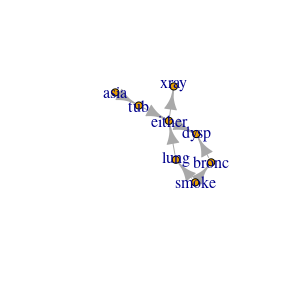This time we specify that no propagation should be performed jt6 <- jt(cp6, propagate = "no") We can now inspect the collecting junction tree and see which cliques are leaves and parents plot(jt6)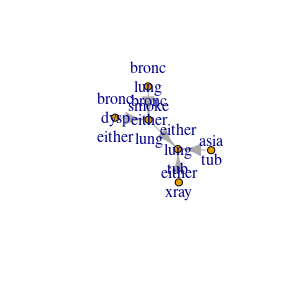get_cliques(jt6) #>$C1
#>  "asia" "tub"
#>
#> $C2 #>  "either" "lung" "tub" #> #>$C3
#>  "bronc"  "either" "lung"
#>
#> $C4 #>  "bronc" "lung" "smoke" #> #>$C5
#>  "bronc"  "dysp"   "either"
#>
#> \$C6
#>  "either" "xray"
get_clique_root(jt6)
#>  "either" "lung"   "tub"
leaves(jt6)
#>  1 4 5 6
unlist(parents(jt6))
#>  2 3 3 2

That is:

• clique 2 is parent of clique 1
• clique 3 is parent of clique 4 etc.

Next, we send the messages from the leaves to the parents

jt6 <- send_messages(jt6)

Inspect again

plot(jt6)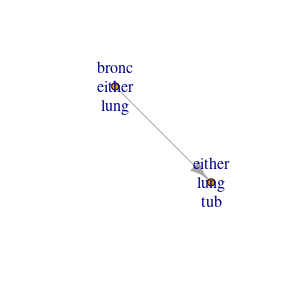Send the last message to the root and inspect

jt6 <- send_messages(jt6)
plot(jt6)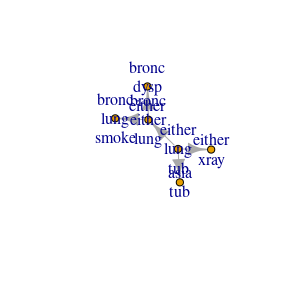The arrows are now reversed and the outwards (distribute) phase begins

leaves(jt6)
#>  2
parents(jt6)
#> []
#>  1 3 6

Clique 2 (the root) is now a leave and it has 1, 3 and 6 as parents. Finishing the message passing

jt6 <- send_messages(jt6)
jt6 <- send_messages(jt6)

Queries can now be performed as normal

query_belief(jt6, c("either", "tub"), "joint")
#>       tub
#> either    yes       no
#>    yes 0.0104 0.054428
#>    no  0.0000 0.935172

## Example 7: Fitting a decomposable model and apply JTA

We use the ess package (on CRAN), found at https://github.com/mlindsk/ess, to fit an undirected decomposable graph to data.

library(ess)
#>
#> Attaching package: 'ess'
#> The following objects are masked from 'package:igraph':
#>
#>     components, dfs, subgraph

g7  <- ess::fit_graph(asia, trace = FALSE)
ig7 <- ess::as_igraph(g7)
cp7 <- compile(pot_list(asia, ig7))
jt7 <- jt(cp7)

query_belief(jt7, get_cliques(jt7)[], type = "joint")
#> , , T = n
#>
#>    L
#> E       n      y
#>   n 0.926 0.0000
#>   y 0.000 0.0652
#>
#> , , T = y
#>
#>    L
#> E       n     y
#>   n 0.000 0e+00
#>   y 0.008 8e-04